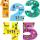# Scale

Cylinder was drawn in scale 2:1. How many times is the volume of the cylinder smaller in reality?

Result

n =  8

#### Solution:Leave us a comment of example and its solution (i.e. if it is still somewhat unclear...):Be the first to comment!## Next similar examples:

1. Garden pondConcrete garden pond has bottom shape of a semicircle with a diameter 1.7 m and is 79 cm deep. Daddy wants make it surface. How many liters of water is in pond if watel level is 28 cm?
2. Total displacementCalculate total displacement of the 4-cylinder engine with the diameter of the piston bore B = 6.6 cm and stroke S=2.4 cm of the piston. Help: the crankshaft makes one revolution while the piston moves from the top of the cylinder to the bottom and back.
3. CylinderThe cylinder surface is 859 dm2, its height is equal to the radius of the base. Calculate height of this cylinder.
4. DonutsFind how many donuts each student will receive if you share 126 donuts in a ratio of 1:5:8If Petra read 10 pages per day, she would read the book two days earlier than she read 6 pages a day. How many pages does a book have?
6. Arble bagA marble bag sold by Rachel's Marble Company contains 5 orange marbles for every 6 green marbles. If a bag has 35 orange marbles, how many green marbles does it contain?
7. Divisible by 5How many three-digit odd numbers divisible by 5, which are in place ten's number 3?
8. CandyPeter had a sachet of candy. He wanted to share with his friends. If he gave them 30 candies, he would have 62 candies. If he gave them 40 candies, he would miss 8 candies. How many friends did Peter have?
9. Waiting roomIn the waiting room are people and flies. Together they have 15 heads and 50 legs (fly has 6 legs). How many people and flies are in the waiting room?
10. Two numbersFind two numbers whose difference and ratio is 2.
11. 1.5 divided1.5 divided by 1 = w divided by 4
12. Unknown numberDetermine the unknown number, which double of its fourth square is equal the fifth its square.
13. EnrollmentThe enrollment at a local college increased 4% over last year's enrollment of 8548. Find the increase in enrollment (x1) and the current enrollment (x2).
14. One halfOne half of ? is: ?
15. CancerOf the 80 people 50 people ill cancer. What percentage of people isn't ill?
16. ShotsDetermine the percentage rate of keeper interventions if from 32 shots doesn't caught four shots.
17. PowersExpress the expression ? as the n-th power of the base 10.